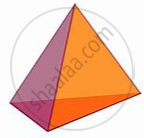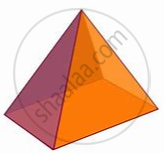# Euler's Formula

#### formula

Euler’s formula ⇒ F + V – E = 2.

# Euler’s formula:

For any polyhedron, F + V – E = 2

Where ‘F’ stands for a number of faces, V stands for a number of vertices and E stands for a number of edges. This relationship is called Euler’s formula.

 Solid Shape F V E F + V E + 2 Cuboid6 8 12 14 14 Triangular Pyramid4 4 6 8 8 Triangular Prism5 6 9 11 11 Pyramid with square base5 5 8 10 10 Prism with square base6 8 12 14 14
If you would like to contribute notes or other learning material, please submit them using the button below.

### Shaalaa.com

What is Euler's Formula? [00:06:44]
S
0%